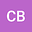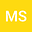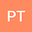Molecular Nitrogen (N2) Density Derived from Optical Measurements During Ionospheric Heating Experiment (or an Alternative Hypothesis for the O(1D) Quenching Coefficient Adjustment).
•••• +2## Abstract

High frequency (HF) experiments inducing intensification of airglow emissions at 6300 Å; and 5577 Å (red and green lines, respectively) from the two lowest excited states of oxygen O(1D) and O(1S) has been studied since the early 1970s. The last generation Arecibo HF facility was commissioned in November 2015, and since then several campaigns have been conducted at AO. The current system consists of six transmitters, each connected to one of six dipole elements, and each capable of continuous wave (CW) operation at a nominal power of ~100 kW. Before the AO platform collapse on December 1, 2020, the HF transmitter system has two available frequencies, 5.125 MHz and 8.175 MHz, with 130 kHz and 100 kHz bandwidths, respectively. In this work we are analyzing the excitation of the red line airglow emission (3600 Å) by high-power radio waves at ~5.125MHz of 28 HF pulses of 5 minutes intercalated by 5 minutes of no HF interaction. The chosen periods were the pre-sunrise and post-sunset periods of June 5, 2016 (Figure 1). Coincidentally, a small geomagnetic storm occurred during these observations. The first experiment started along the initial phase of this disturbance and the second experiment at the end of the main phase (Figure 2). Up to now, our main findings are listed below: 1. Assuming that the modified red line comes from a narrow height range in the vicinity of the reflection height to a first approximation and considering that all of the excess emission comes from a single height (equation 1) (which corresponds to the height where the plasma frequency equals the transmitted frequency), it was detected that the lifetime of the O(1D) varies with altitude which a peak close to the red line emission altitudes (Figure 3). Θ_r=1/((T1+T2+T3+T4)) (1) Where T1 is the total Einstein transition probability of the O(1D) state, T2 is the N2 concentration at the altitude of reflection times its respective quenching coefficient (Q~5,0.10-11cm3s-1) as well as T3 the O2 concentration times its respective quenching coefficient (R~7,4.10-11cm3s-1). 2. Assuming a fixed lifetime for all altitudes, we detected variation of the N2 quenching coefficient O(1D) also varying with altitude. Such variation could be a miss determination of the N2 neutral concentration from the NRLMSISE-00 Atmosphere Model (Figure 4) (equation 1). 3. As a practical outcome, our study shows that the 5 minutes off is not sufficient for the excited region to return to the previous quiet condition. Our computations show that pulses of 3 minutes intercalated by 6 minutes off are the ones more appropriate (Figure 5).